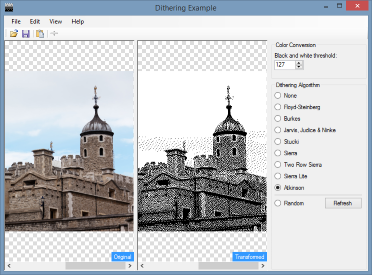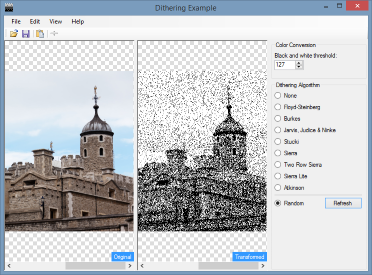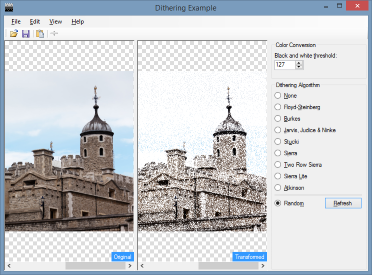## Even more algorithms for dithering images using C#

Although I should really be working on adding the dithering algorithms into Gif Animator, I thought it would be useful to expand the repertoire of algorithms available for use with it and the other projects I'm working on.

### Adding a general purpose base class

I decided to re-factor the class I created for the Burkes algorithm to make it suitable for adding other error diffusion filters with a minimal amount of code.

First, I added a new abstract class, `ErrorDiffusionDithering`. The constructor of this class requires you to pass in the matrix used to disperse the error to neighbouring pixels, the divisor, and whether or not to use bit shifting. The reason for the last parameter is the Floyd-Steinberg and Burkes algorithms covered in my earlier posts had divisors that were powers of two, and so could therefore be bit shifted for faster division. Not all algorithms use a power of two divisor though and so we need to be flexible.

The constructor then stores the matrix, and pre-calculates a couple of other values to avoid repeating these each time the `Diffuse` method is called.

``````protected ErrorDiffusionDithering(byte[,] matrix, byte divisor, bool useShifting)
{
if (matrix == null)
{
throw new ArgumentNullException("matrix");
}

if (matrix.Length == 0)
{
throw new ArgumentException("Matrix is empty.", "matrix");
}

_matrix = matrix;
_matrixWidth = (byte)(matrix.GetUpperBound(1) + 1);
_matrixHeight = (byte)(matrix.GetUpperBound(0) + 1);
_divisor = divisor;
_useShifting = useShifting;

for (int i = 0; i < _matrixWidth; i++)
{
if (matrix[0, i] != 0)
{
_startingOffset = (byte)(i - 1);
break;
}
}
}``````

The actual dithering implementation is unchanged from original matrix handling code, with the exception of supporting bit shifting or integer division, and not having to work out the current pixel in the matrix, width or height.

``````void IErrorDiffusion.Diffuse(ArgbColor[] data, ArgbColor original, ArgbColor transformed, int x, int y, int width, int height)
{
int redError;
int blueError;
int greenError;

redError = original.R - transformed.R;
blueError = original.G - transformed.G;
greenError = original.B - transformed.B;

for (int row = 0; row < _matrixHeight; row++)
{
int offsetY;

offsetY = y + row;

for (int col = 0; col < _matrixWidth; col++)
{
int coefficient;
int offsetX;

coefficient = _matrix[row, col];
offsetX = x + (col - _startingOffset);

if (coefficient != 0 && offsetX > 0 && offsetX < width && offsetY > 0 && offsetY < height)
{
ArgbColor offsetPixel;
int offsetIndex;
int newR;
int newG;
int newB;

offsetIndex = offsetY * width + offsetX;
offsetPixel = data[offsetIndex];

// if the UseShifting property is set, then bit shift the values by the specified
// divisor as this is faster than integer division. Otherwise, use integer division

if (_useShifting)
{
newR = (redError * coefficient) >> _divisor;
newG = (greenError * coefficient) >> _divisor;
newB = (blueError * coefficient) >> _divisor;
}
else
{
newR = (redError * coefficient) / _divisor;
newG = (greenError * coefficient) / _divisor;
newB = (blueError * coefficient) / _divisor;
}

offsetPixel.R = (offsetPixel.R + newR).ToByte();
offsetPixel.G = (offsetPixel.G + newG).ToByte();
offsetPixel.B = (offsetPixel.B + newB).ToByte();

data[offsetIndex] = offsetPixel;
}
}
}
}``````

### Burkes Dithering, redux

The `BurkesDithering` class now looks like this

``````public sealed class BurksDithering : ErrorDiffusionDithering
{
public BurksDithering()
: base(new byte[,]
{
{
0, 0, 0, 8, 4
},
{
2, 4, 8, 4, 2
}
}, 5, true)
{ }
}``````

No code, just the matrix and the bit shifted divisor of 5, which will divide each result by 32. Nice!

### More Algorithms

As well as opening the door to allowing a user to define a custom dither matrix, it also makes it trivial to implement a number of other common error diffusion matrixes. The GitHub Repository now offers the following algorithms

• Atkinson
• Burkes
• Floyd-Steinberg
• Jarvis, Judice & Ninke
• Sierra
• Two Row Sierra
• Sierra Light
• Stucki

Which is a fairly nice array.``````public sealed class AtkinsonDithering : ErrorDiffusionDithering
{
public AtkinsonDithering()
: base(new byte[,]
{
{
0, 0, 1, 1
},
{
1, 1, 1, 0
},
{
0, 1, 0, 0
}
}, 3, true)
{ }
}``````

### Random Dithering

There's a rather old (in internet terms anyway!) text file floating around named DHALF.TXT (based in turn on an even older document named `DITHER.TXT`) that has a ton of useful information on dithering, and with the exception of the Altkinson algorithm (I took that from here) is where I have pulled all the error weights and divisors from.

One of the sections in this document dealt with random dithering. Although I didn't think I would ever use it myself, I thought I'd add an implementation of it anyway to see what it's like.

Unlike the error diffusion methods, random dithering affects only a single pixel at a time, and does not consider or modify its neighbours. You also have a modicum of control over it too, if you can control the initial seed of the random number generator.

The `DHALF.TXT` text sums it up succinctly: For each dot in our grayscale image, we generate a random number in the range 0 - 255: if the random number is greater than the image value at that dot, the display device plots the dot white; otherwise, it plots it black. That's it.

And here's our implementation (ignoring the fact that it isn't error diffusion and all of a sudden our `IErrorDiffusion` interface is named wrong!)

``````void IErrorDiffusion.Diffuse(ArgbColor[] data, ArgbColor original, ArgbColor transformed, int x, int y, int width, int height)
{
byte gray;

gray = (byte)(0.299 * original.R + 0.587 * original.G + 0.114 * original.B);

if (gray > _random.Next(0, 255))
{
data[y * width + x] = _white;
}
else
{
data[y * width + x] = _black;
}
}``````

(Although I reversed black and white from the original description as otherwise it looked completely wrong)I was surprised to see it actually doesn't look that bad.

### Continuation

I've almost got a full house of useful dithering algorithms now. About the only thing left for me to do is to implement a ordered Bayer dithering as I really like the look of this type, and reminds me of games and computers of yesteryear. So there's still at least one more article to follow in this series!

The updated source code with all these algorithms is available from the GitHub repository.